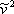# 9.1: Introduction

•• Vice Provost Emeritus for Global Program & Professor Emeritus (Petroleum and Natural Gas Engineering) at Pennsylvania State University
• Sourced from John A. Dutton: e-Education Institute

If we multiply the vdW EOS (expression 7.11a) byand expand the factorized product by applying the distributive law, the result is the vdW EOS expressed in terms of molar volume, as follows:

$\bar{v}^{3}-\left(b+\frac{R T}{P}\right) \bar{v}^{2}+\left(\frac{a}{P}\right) \bar{v}-\frac{a b}{P}=0 \label{9.1}$

Note that Equation \ref{9.1} is a third order polynomial in $$\bar{v}$$ i.e., it is cubic in molar volume. Additionally, we can substitute the definition of compressibility factor $$Z$$,

$Z=\frac{P \bar{v}}{R T} \label{9.2}$

into Equation \ref{9.1} and obtain a different cubic polynomial in $$Z$$, as shown:

$Z^{3}-\left(1+\frac{b P}{R T}\right) Z^{2}+\left(\frac{a P}{R^{2} T^{2}}\right) Z-\frac{a b P^{2}}{(R T)^{3}}=0 \label{9.3}$

As we see, vdW EOS is referred to as cubic because it is a polynomial of order 3 in molar volume (and hence in compressibility factor $$Z$$). In general, any equation of state that is cubic in volume (and $$Z$$) and explicit in pressure (Equation 7.11b) is regarded as a cubic equation of state. vdW EOS is a cubic EOS, and all the transformations and modifications that it has undergone during the more than one hundred years since its publication are also cubic EOS; or better, they are in-the-van-der-Waals-spirit EOS or of-the-van-der-Waals-family EOS.